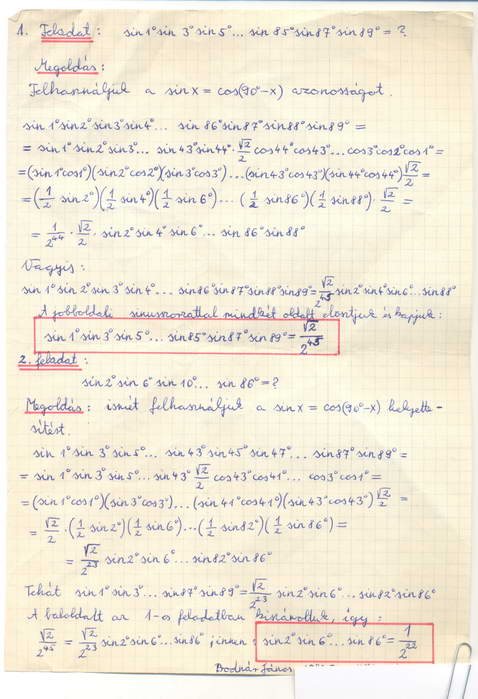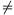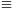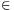Középiskolai Matematikai és Fizikai Lapok
Informatika rovattal
 Már regisztráltál? Új vendég vagy?

Fórum: "ujjgyakorlatok"

                                                                                                                                                    

 Szeretnél hozzászólni? Jelentkezz be. 10 hozzászólás/oldal 25 hozzászólás/oldal 50 hozzászólás/oldal 100 hozzászólás/oldal 200 hozzászólás/oldal időben csökkenő időben növekvő
  juantheron 2012-05-25 14:44:28Thanks sakkmath Two tasks (1) last 3 digits ofRóbert Gida 2012-05-22 20:46:03Úgy már igaz lehet. (n=3-ra jónéhány esetet géppel megnéztem.) Előzmény:  Csimby, 2012-05-22 20:39:10
  Csimby 2012-05-22 20:39:10és ha n>2? Előzmény:  Róbert Gida, 2012-05-22 20:36:16
  Róbert Gida 2012-05-22 20:36:16Legyen n=2;a1=1;a2=2, ekkor a két oldal egyenlő, de az ai-k nem egyenlőek. Előzmény:  Csimby, 2012-05-22 20:27:55
  Csimby 2012-05-22 20:27:55Utolsó próbálkozás: Legyen n2, ai-k mint előbb, ekkor:egyenlőség acsa ha ai-k egyenlőek. Előzmény:  Csimby, 2012-05-22 18:43:47
  Róbert Gida 2012-05-22 19:40:14Ez pedig n=3;a1=a2=a3=2-re nem teljesül. Előzmény:  Csimby, 2012-05-22 18:43:47
  Csimby 2012-05-22 18:43:47Na szóval, legyenek a1,a2...,an poz. valósak, továbbá n3 Biz.be:egyenlőség pontosan akkor ha a1=...=an. Előzmény:  Csimby, 2012-05-22 18:12:38
  Csimby 2012-05-22 18:33:21Bocs, a bal oldalt szumma van!! Előzmény:  Róbert Gida, 2012-05-22 18:23:32
  Róbert Gida 2012-05-22 18:23:32n=2;a1=a2=8-re nem teljesül az egyenlőtlenség. Előzmény:  Csimby, 2012-05-22 18:12:38
  Csimby 2012-05-22 18:12:38Legyenek a1,a2,...,an pozitív valós számok. Biz. be:Egyenlőség pontosan akkor ha a1=a2=...=an.
  sakkmath 2012-05-19 16:10:25Az utoljára felvetett kérdésben csak idáig jutottam: cot (1°).cot (2°).cot (3°).....cot (43°).cot (44°)=tan (46°).tan (47°).tan (48°).....tan (87°).tan (89°) Ha valaki megkapta volna a szorzat számértékét, kérem, ne tartsa titokban :-) Előzmény:  juantheron, 2012-05-18 17:02:03
  sakkmath 2012-05-19 00:25:39This nostalgia for me: About 30 years ago, I proposed to publish these two problems. (Not published them ...)Előzmény:  sakkmath, 2012-05-18 15:35:51
  juantheron 2012-05-18 17:02:03Thanks Sakkmath i have got my solution after seeing your Answer bcz I have solve a simillar Problem Yesterday where I have calculate value of sin (10).sin (20).sin (30)......sin (890) Now I have another doubt in my min can we calculate the value of cot (10).cot (20).cot (30)........cot (440)=
  juantheron 2012-05-18 16:58:09Let A=cos (10).cos (20).cos (30).........cos (890) Now using cos (90-a)=sin (a) So A=sin (10).sin (20).sin (30).........sin (890) and Let B=sin (20).sin (40).sin (60).........sin (880) Now AB=(sin (10).sin (890)).(sin (20).sin (880)).........(sin (440).sin (460)).sin (450) So AB=(sin (10).cos (10)).(sin (20).cos (20)).........(sin (440).cos (440)).sin (450) SoSoSobcz B0
  juantheron 2012-05-18 16:57:24Thanks sakkmath after seeing your answer I have got a method bcz i have solve a simillar problem Yesterday where i have calculate the value of sin (10).sin (20).sin (30).........sin (890) My solution:: Let A=cos (10).cos (20).cos (30).........cos (890) Now using cos (90-a)=sin (a) So A=sin (10).sin (20).sin (30).........sin (890) and Let B=sin (20).sin (40).sin (60).........sin (880) Now AB=(sin (10).sin (890)).(sin (20).sin (880)).........(sin (440).sin (460)).sin (450) So AB=(sin (10).cos (10)).(sin (20).cos (20)).........(sin (440).cos (440)).sin (450) SoSoSobcz B0
  sakkmath 2012-05-18 15:35:51The solution:For details, let me quote a little later. Előzmény:  juantheron, 2012-05-18 07:06:44
  juantheron 2012-05-18 07:06:44Thanks friends got it. New task:: Numerical value of cos (10).cos (30).cos (50).......cos (890)= where all angle are in Degree
  m2mm 2012-05-17 21:16:33We may assume that xy. Then (y+2)2=y2+4y+4>y2+3x>y2. So, if y2+3x is a square, it must be (y+1)2. Then y2+2y+1=y2+3x, so 2y+1=3x, which means that 2y-1 (mod 3), so y1 (mod 3). Hence, y=3k+1 for some positive integer k. Since 2y+1=3x, then 6k+3=3x, 2k+1=x. As x2+3y is a square, then (2k+1)2+3(3k+1)=4k2+4k+1+9k+3=4k2+13k+4 as well. But (2k+2)2=4k2+8k+44k2+13k+4<4k2+16k+16=(2k+4)2, so 4k2+13k+4=(2k+2)2=4k2+8k+4, or 4k2+13k+4=(2k+3)2. If 4k2+13k+4=4k2+8k+4, then k=0, x=y=1 (and this pair is a soultion). If 4k2+13k+4=(2k+3)2, then 4k2+13k+4=4k2+12k+9, so k=5. Then the solution is (11,16) or (16,11). Előzmény:  juantheron, 2012-05-16 20:17:02
  logarlécész 2012-05-17 20:25:55Teljesen igaz. Hiába, a figyelmetlenség mindig játszik. :-) Előzmény:  nadorp, 2012-05-16 14:16:10
  nadorp 2012-05-17 14:18:36"(x-y).(x+y-3)=(l+m).(l-m) means either x+y-3=l+m and x-y=l-m " Incorrect. For example 3.8=6.4 but 86 and 34 Előzmény:  juantheron, 2012-05-17 08:49:02
  juantheron 2012-05-17 08:49:02Thanks Nadorp got it task:: find positive Integer value of x and y for which x2+3y and y2+3x is an perfect square My solution:: Let x2+3y=l2 and y2+3x=m2 Where l,mZ Then (x2-y2)-3(x-y)=l2-m2 (x-y).(x+y-3)=(l+m).(l-m) means either x+y-3=l+m and x-y=l-m 2x=3+2l orsimilarlybut when i put rendomly put values like (1,1) and (11,16) and (16,11) but how can i calculate it Thanks
  nadorp 2012-05-17 08:23:48"But Not sure about k7 has no value for which satisfy the given equation" Answer: x2=4+[x]4+x x2-x-40Előzmény:  juantheron, 2012-05-16 19:46:00
  juantheron 2012-05-16 20:17:02Nwe task:: The number of ordered pairs of positive integers x,y such that x2+3y and y2+3x are both perfect squares
  juantheron 2012-05-16 19:46:00Yes Friends your answer is Right I have solved like that way x2=4+[x] here R.H.S(right hand side) is an Integer that means L.H.S(Left hand side must be an Integer) So x2Z So Let x2=k, where k>0 SoSo our equation convert intoNow after checking certain value of k like k=1,2,3,4,5,6 We Get only k=2 and k=6 satisfy the given equation and after k7, we get L.H.S # R.H.S But Not sure about k7 has no value for which satisfy the given equation can anyone clear last line explanation Thanks
  juantheron 2012-05-16 19:35:38Thanks Nadorp and Small Potato for Integration I have solve it after yours work I have solved it like in that wayPut (cos x-sin x)=tand (sin x+cos x)dx=-dt andafter that we can calculate easily